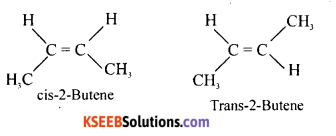# 1st PUC Chemistry Model Question Paper 2 with Answers

Students can Download 1st PUC Chemistry Model Question Paper 2 with Answers, Karnataka 1st PUC Chemistry Model Question Papers with Answers helps you to revise the complete Karnataka State Board Syllabus and score more marks in your examinations.

## Karnataka 1st PUC Chemistry Model Question Paper 2 with Answers

Time: 3.15 Hours
Max Marks: 70

Instruction:

1. The questions paper has five parts A, B, C, D and E. All parts are compulsory.
2. Write balanced chemical equations and draw labeled diagram wherever allowed.
3. Use log tables and simple calculations f necessary (use of scientific calculations is not allowed).

Part – A

I. Answer ALL of the following (each question carries one mark): ( 10 × 1 = 1 )

Question 1.
Express 0.001023 into scientific notation.
1.023 x 10-3.

Question 2.
Define critical temperature.
The temperature above which gas cannot be liquified.

Question 3.
Give the example which acts as Lewis base as well as Bronsted base.
NH3 or Ammonia.Question 4.
How does electronegativity related to atomic size?
Atomic size ∝ $$\frac{1}{\text { Electronegativity }}$$
Electronegativity

Question 5.
What is the oxidation state of oxygen in peroxide?
-1 or minus 1

Question 6.
Which alkali metal is act as strong reducing agent?
Lithium.

Question 7.
Write the formula of inorganic benzene.
B3N3H3

Question 8.
What is dry ice?
Solid CO2.Question 9.
Complete the reaction NH4CNO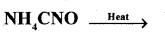Question 10.
Write the name of the chain isomer of n-Butane.
CH3-CH2-CH2-CH3

Part – B

II. Answer any FIVE of the following questions carrying TWO marks: ( 5 × 2 = 10 )

Question 11.
Calculate the average atomic mass of chlorine using the following data.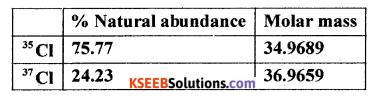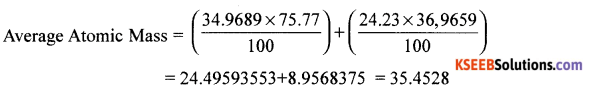Question 12.
Draw the graph of pressure versus volume of a gas at a different temperatures to illustrate the Boyle’s law.\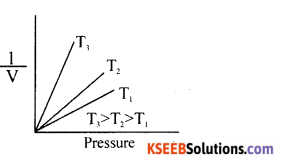Question 13.
Give any two conditions for hybridization of atomic orbitals.
(i) Should contain half filled atomic orbitals.
(ii) Energy of the combining atomic orbitals should be equivalent.

Question 14.
Explain the reactivity of second group elements towards hydrogen.
Hydrogen combines with second group elements to form metallic hydrides,
i.e. M + H2 → MH2
OR
Ca + H2 → CaH2
when M = Mg, Ca, Ba, etc.Question 15.
How many sigma and pi bonds are in carbon monoxide?
2σ bonds and one 7t-bond.

Question 16.
Write the structural isomers of an alkene with molecular formula C2H8.
H3C-H2C-HC=CH2 , CH3-CH=CH-CH3

Question 17.
What are the characteristics for any ring system to be called as aromatic compound?
(i) It should be planar.
(ii) Obeys Huckel rule i.e. (4n + 2)π electrons.
(iii) Should contain delocalized π – electrons above and below the plane of the molecule.

Question 18.
(a) Which oxide of nitrogen in higher concentration will retard the rate of photosynthesis in plants?
NO2 or Nitrogen dioxide.
(b) Name the compound formed when carbon monoxide binds to haemoglobin.
Carboxyhaemoglobin (Hb + CO → HbCO).

Part – C

III. Answer any FIVE of the following questions carrying THREE marks: ( 5 × 3 = 15 )

Question 19.
Why Beryllium exhibit anomalous behaviour from the rest of the elements in the group.
Due to

1. Smaller size compared to other elements in the group.
2. High ionisation enthalpy in the ground state.

Question 20.
With the help of hybridization explain the structure of methane.
CH4
EC of C = 1s22s22px12py12pz1
The four half filled orbitals overlaps with each other giving 4sp3 hybrid orbitals. These combines with s-orbital of hydrogen along the axis giving 4σ bonds with bond angle 109°28′ and tetraheral geometry.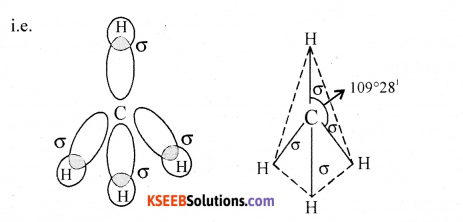Question 21.
(a) What is bond enthalpy? How it is related to the bond order?

1. The amount of energy required to break one mole of bonds of same type to separate them into gaseous atoms.
2. Bond enthalpy oc Bond order.

(b) Write the resonance structure of CO2.Question 22.
(a) Give any two differences between bonding and anti-bonding molecular orbitals.
BMO :

1. Formed by addition overlapping ΨAB = ΨA + ΨB
2. Less energy than ABMO.
3. More stable.

ABMO :

1. Formed by the subtraction overlapping of atomic orbitals. of atomic orbitals i.e. ΨAB = ΨAB
2. More energy than BMO.
3. Less stable.

(b) What is the dipole moment of BeF2?
Dipole moment is 0 (zero)
F ⇌ Be ⇌ FQuestion 23.
Balance the following redox reaction by half reaction method.
MnO4 (aq) + 1 (aq) → MnO2 (s) + I2(s) : Basic medium.Question 24.
Give the reactions to show amphoteric nature of water.(ii) Mention any one method of removal of temporary hardness of water.
By boiling.Question 25.
How caustic soda is commercially prepared from brine by Castner-Kellner cell.
Caustic soda by Castner-Kellner cell: NaOH is manufactured by the electrolysis of aqueous solution of NaCl (Brine).
i.e. 2NaCl → 2Na+ + 2Cl
Sodium ions are discharged at mercury cathode.
Sodium deposited at mercury forms sodium amalgam.
Chlorine liberated at anode removed from the cell.
At cathode : 2Na+ + 2e → Na, Na + Hg → Na – Hg
At anode : 2Cl – 2e → Cl2
Na-Hg is treated with water to form NaOH
i.e. Na/Hg + 2H2O → 2NaOH + H2 + Hg.

Question 26.
Give the example of element of group 14
(i) Shows maximum catenation capacity.
Carbon

(ii) Used as semiconductor.
Silicon

(iii) Which reacts with water.
Tin

Part – D

IV. Answer any FIVE of the following questions carry ing FIVE marks: ( 5 x 5 = 25 )

Question 27.
(a) M atch the following :(i)-(c),
(ii)-(d),
(iii)-(a)

(b) Define molarity. Write the expression to calculate the molarity of the solution for the given mass and volume.
It is the number of moles of solute present in 1000 ml solvent or 1dm3.
M = $$\frac{\mathrm{W}}{\mathrm{GMM}} \times \frac{1000}{\mathrm{V}}$$

Question 28.
(a) Give any three posulates of Bohr’s model for hydrogen atom.

• Electrons are revolving around nucleus in a closed circular path called orbits or main shells or energy levels.
• When an electron jumped from higher energy level to lower energy level, the difference of energy emitted as radiation, i.e. E2 – E1 = ∆E= hγ
• The angular momentum of an electron has discrete values. It is given by the equation
mvr = $$\frac{n h}{2 \pi}$$

(b) Calculate the mass of a photon with wavelength 5,OA°Question 29.
(a) Sketch the shapes of Px and dz2.(b) Identify the property exhibited by 19K40 and 19Ca40.
Isobars (∵ mass number are equal).

(c) Write the orbital (box) type electronic configuration of p4 and d4 according to Hund’s rule of maximum of multiplicity.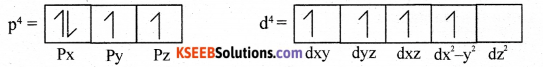Question 30.
a) Derive ideal gas equation using gas laws.
Ideal gas equation:
According to Boyle’s law V ∝ $$\frac{1}{P}$$ T at constant T
According to Charle’s law V ∝ at constant P
According to Avogadro’s law V ∝ n at constant T and P
On coming V ∝ $$\frac{1}{P}$$ V ∝ $$\frac{1}{P}$$ x T x n
or PV=nRT
For ‘n’ moles, PV = RT for 1 mole.
R = gas constant, T = Kelvin Temp, P = Pressure, V = Volume of gas
n = Number of moles of gas.

(b) At 25°C and 760 mm of Hg pressure a gas occupies 600 mL volume. What will be its pressure at a height where temperature is 10°C and volume of the gas is 640 ml.
Initial conditions
P1 = 760 mm
V1 = 600 mm
T1 = 250C = 298K

Final conditions
P 2= ?
v2 = 640 mL
T2= 10°C + 273k = 283KQuestion 31.
(a) If water vapour is assumed to be a perfect gas, molar enthalpy change for vapourization of 1 mole of water at 1 bar ad 100°C is 41kJ mole-1. Calculate the internal energy change when 1 mole of water is vapourized at 1 bar pressure and 100°C.
H2O (l) → H20 (g)
∆ng = 1 – 0 = 1
W.K.T. LH = ∆U + ∆ngRT
OR ∆U = ∆H – ∆ngRT = 41 x 103 – 1 x 8.314 x 373
= 41000— 3101,122 = 37898.878 J/mol
OR = 37.898878 K/J/mol.

(b) State Hess’s law of constant heat summation.
The total amount of heat liberated or absorbed is same whether the reaction takes place in one step or more than one step / (or several steps).

(c) What is the value for standard enthalpy of formation of an element.
Zero/0

Question 32.
(a) Calculate ∆G° for conversion of oxygen to ozone, -O2(g) —> O3(g) at 298K. If
Kp for this conversation is 2.47 x 10-29.
∆rG° = ? R = 8.314J/k/mol T = 298K Kp = 2.47 x 10-29.
W.K.T. ∆rG° = -2.303RTlogKp = 2.303x 8.314 x 298 x 2.47 x 10-29.
= 163229 J/mol or 163.2 KJ/mol.

(b) What is thermochemical equation? Write the thermochemical equation for the molar combustion of ethanol (Given ∆rH° = -1367 kJ mol-1).

1. It is a balanced reaction, gives the information about physical state of reactants and product as well as heat liberated or absorbed.
2. C2H5OH(l)+ 3O2(g) → 2CO2(g) + 3H20(/) ; ∆H° = -1367 KJ/mol

(c) What is the value of ∆G in a spontaneous process?
∆G = -veQuestion 33.
For the equilibrium 2NOCl (g) ⇌ 2NO(g) + Cl2(g) the value of the equilibrium constant Kc is 3.75 x 10-6 at 1069K. Calculate the Kp for the reaction at this temperature?
For the reaction 2NOCl (g) ⇌ 2NO(g) + Cl2(g)
∆n = 3 – 2 = 1. ∴ Kp = Kc (RT)∆n
Kp = (3.75 x 10-6 x 0.0831 x 1069) x 1 = 3.75 x 0.0831 x 1069 x 10-6 = 333.13 x 10-6 OR Kp= 3.33 x 10-4

(b) Write any two general characteristics of equilibria involving physical process.

1. It is dynamic in nature.
2. It can be achieved in a closed vessel.
3. It depends only on temperature but not on concentration or pressure.

Question 34.
(a) The pH of the blood is 7.4. Calculate the [H+].
pH = 7.4 [H+] = ?
W.K.T [H+] = Antilog (-pH)
[H+]= Antilog 10 (-7.4)
Add-1 to-7 and +1 to-0.4 ∴ [H+] = Antilog $$\overline{8.6}$$
[H+] = 10-8 x Antilog of (+0.6).

(b) Derive the Henderson-Hasselbalch equation for acid buffer.
Henderson-Hasselbalch equation:
Consider a weak acid and its salt BA,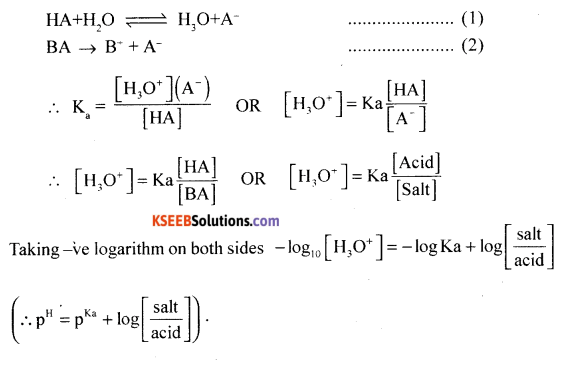Part – E

V. Answer any TWO of the following questions carrying FIVE marks: ( 2 x 5 = 10 )

Question 35.
(a) For the following bond cleavage, use curved arrows to show electron flow, mention the type of bond cleavage, and reactive intermediate formed.
CH3CH2O + OCH2CH3 → CH3CH2O + OCH2CH3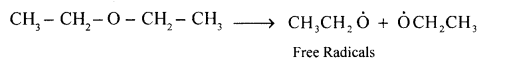1. Homolytic cleavage

(b) Give the hybridisation and geometry of carbocation.
sp2 and planar.Question 36.
(a) 0.2033 g of an organic compound on combustion gave 0.3780 g of CO2 and 0.1288 g H2O. In a separate experiment 0.1877 g of the compound on analysis by Dumas method produced 31.7 ml. of nitrogen collected over water at 14°C and 758 mm pressure. Determine the percentage composition of the compound. (Aqueous tension of water at 14°C = 12 mm pressure).
Given mass of organic compound = 0.2033 g |
Volume of nitrogen (V1) = 31.7 mL
T1 = 287K P1 = (P – f) = 758 – 12 = 746 mm;
P2 = 760mm U2 = ? T2 = 273K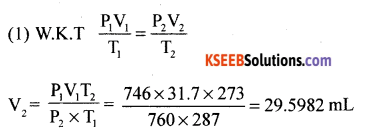To convert the volume of of N2 at STP into mass
22400 mL of nitrogen at STP weighs = 28 g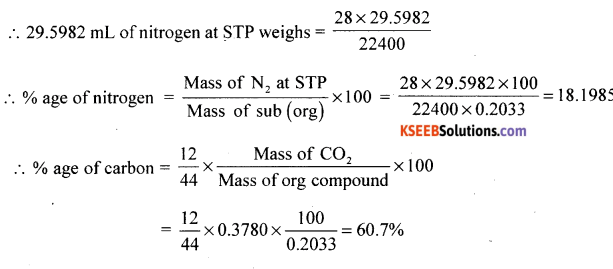% age of oxygen = 100 – (18.1985 + 50.71 + 7.03) = 24.06%

(b) What are nucleophiles? Give an example.
The negatively charged species reacts at nucleus or +ve centre are called nucleophiles.
Example: Cl, Br, I, $$\mathrm{C} \overline{\mathrm{N}}$$, NH3, etc.

Question 37.
(a) Explain the mechanism of addition of HBr to propene in the presence of peroxide catalyst.
It is a free radical mechanism.
It has the following steps:
(a) Initiation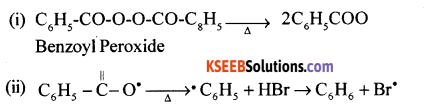(b) Propagation(c) Termination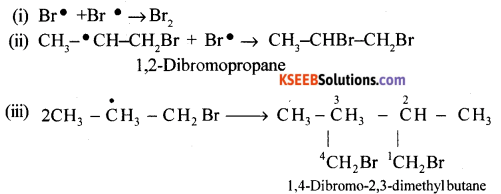(b) Write the structures of cis and trans isomers of But-2-ene.